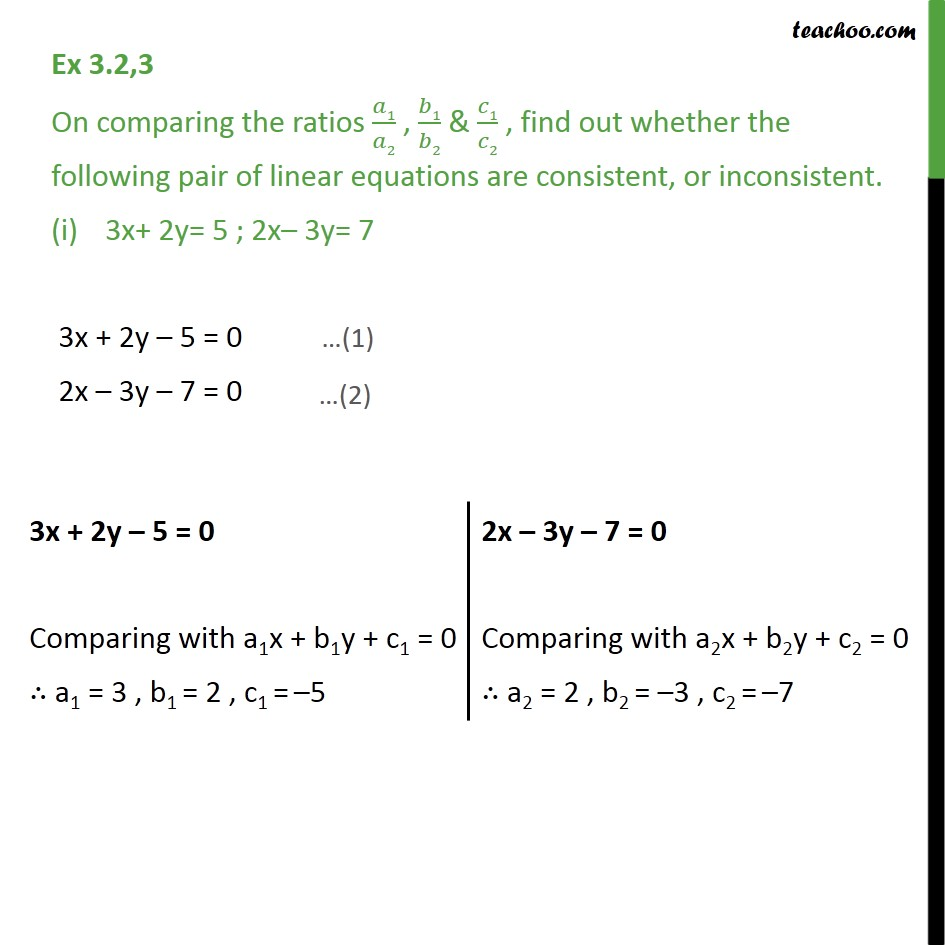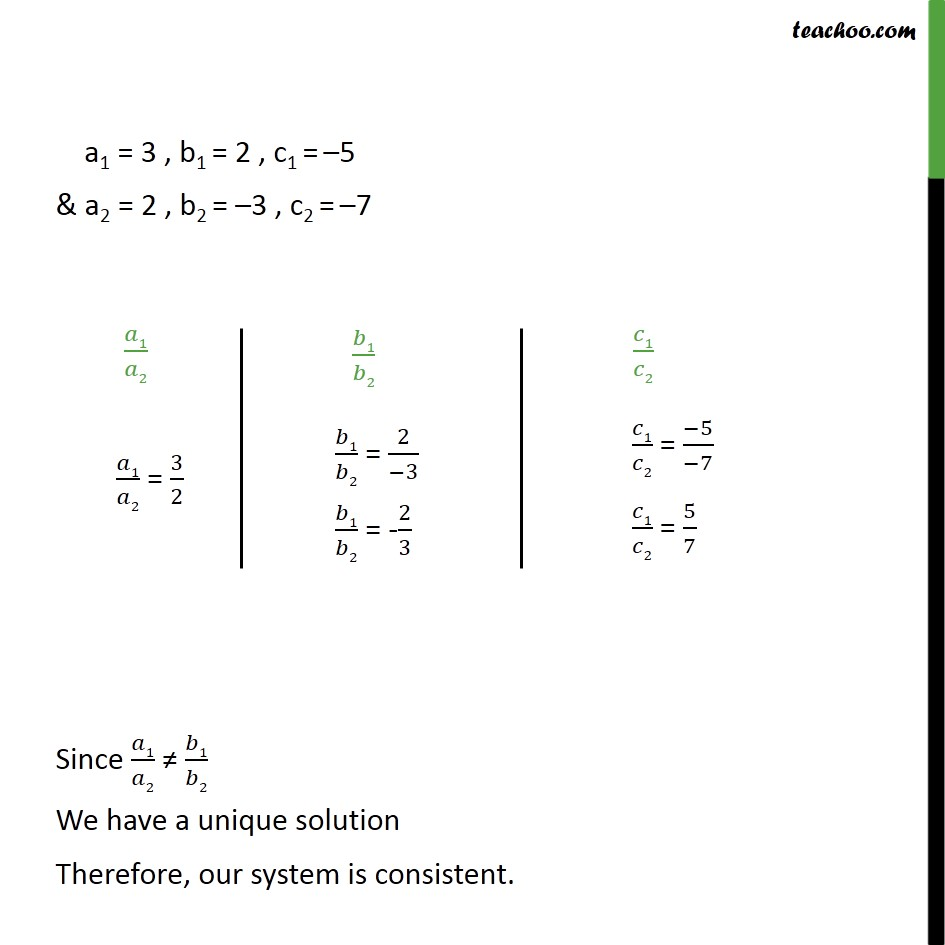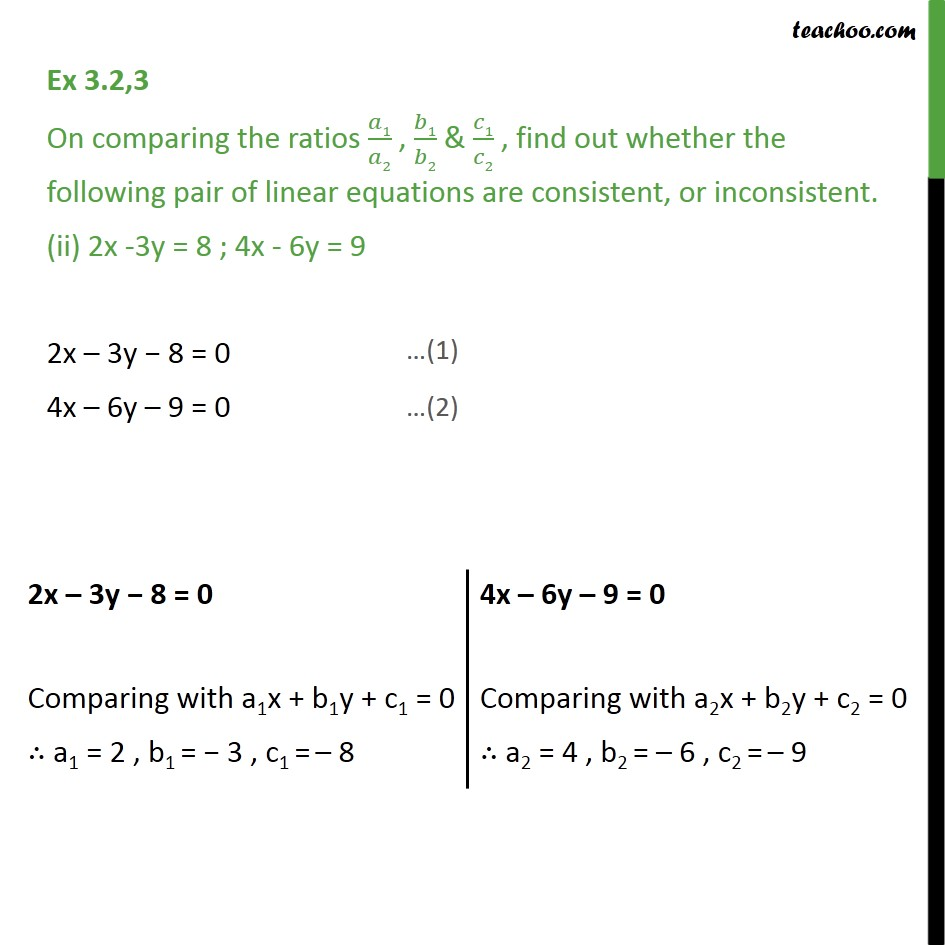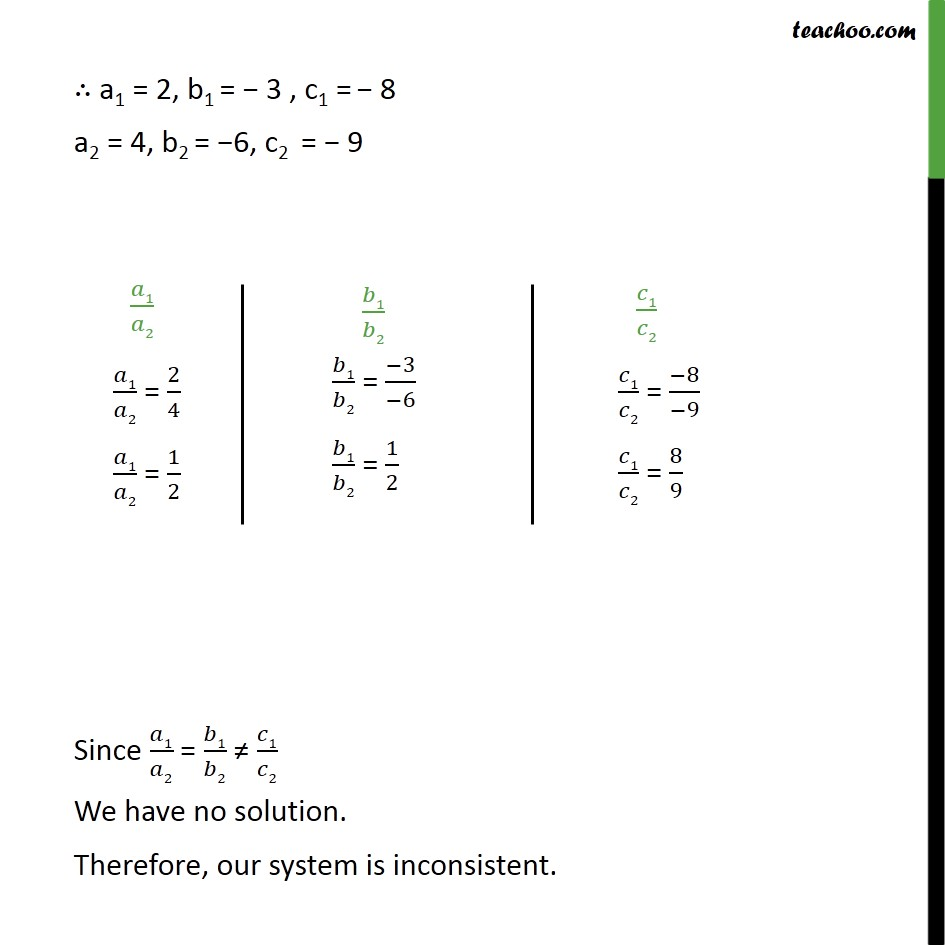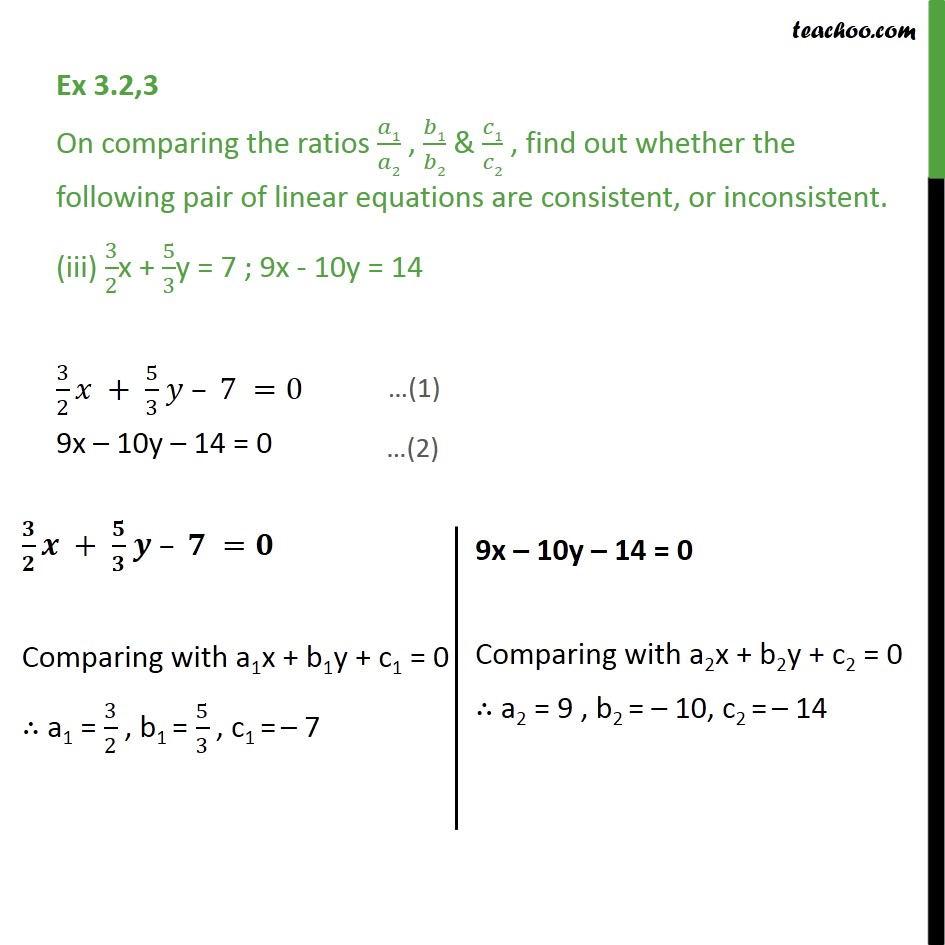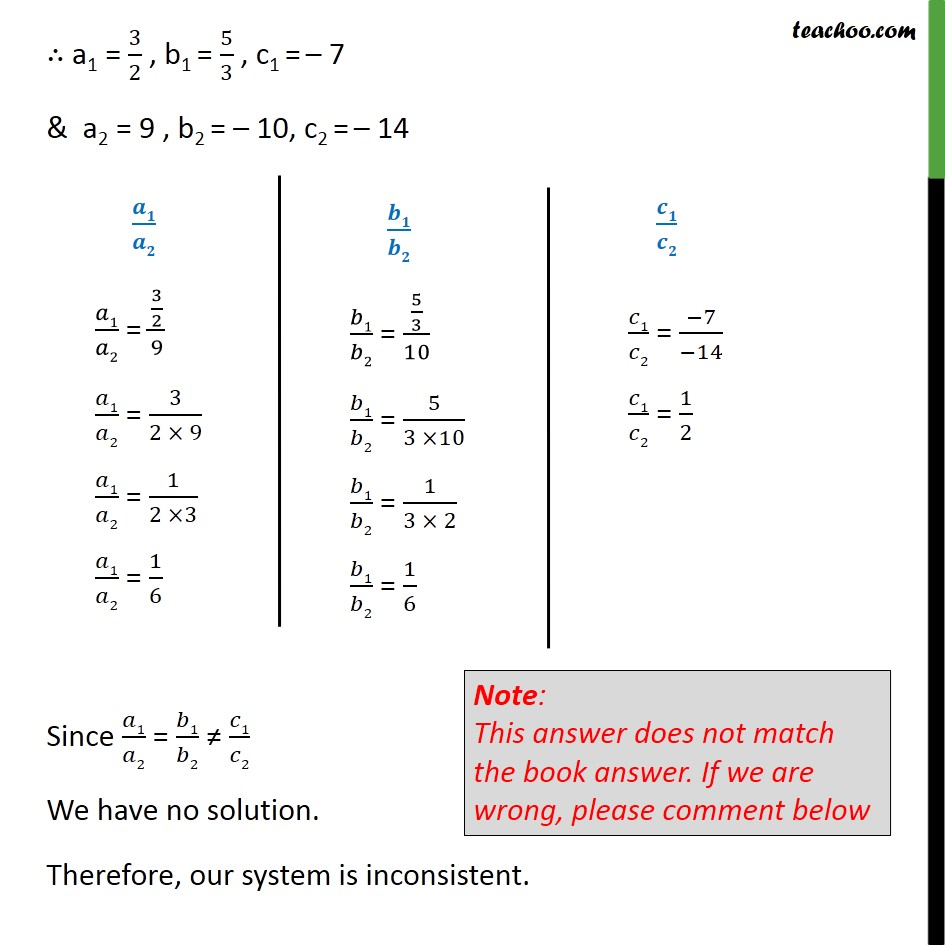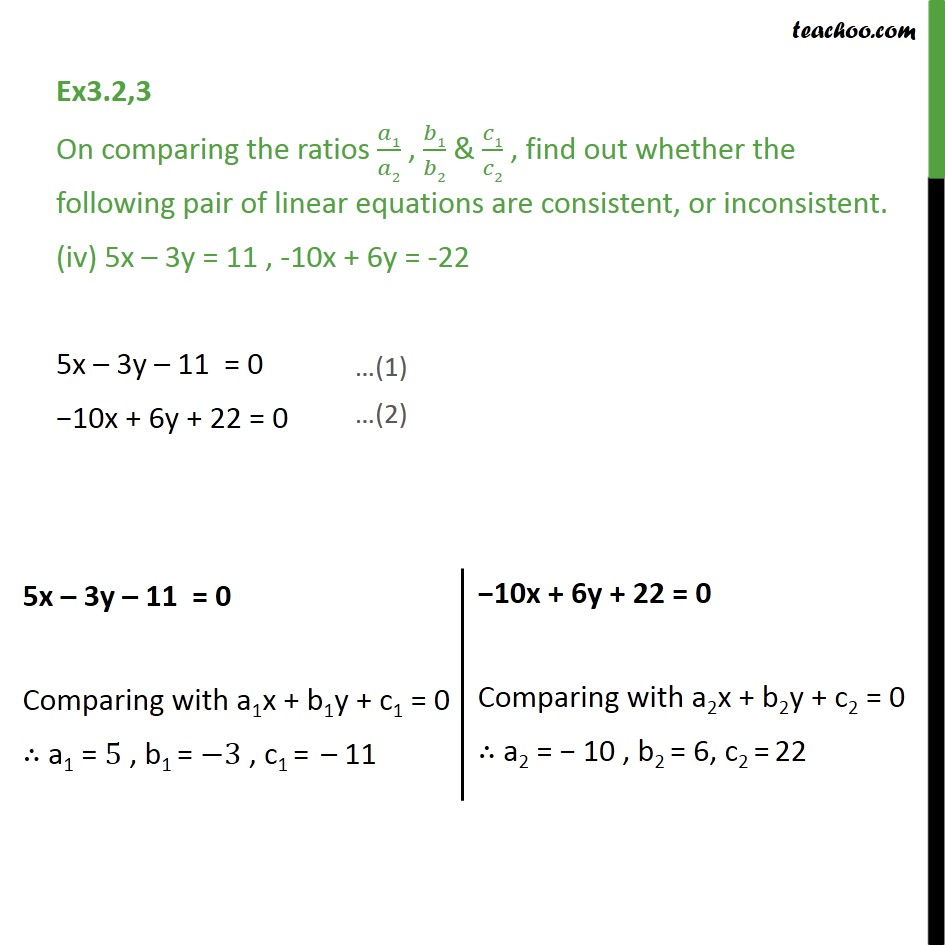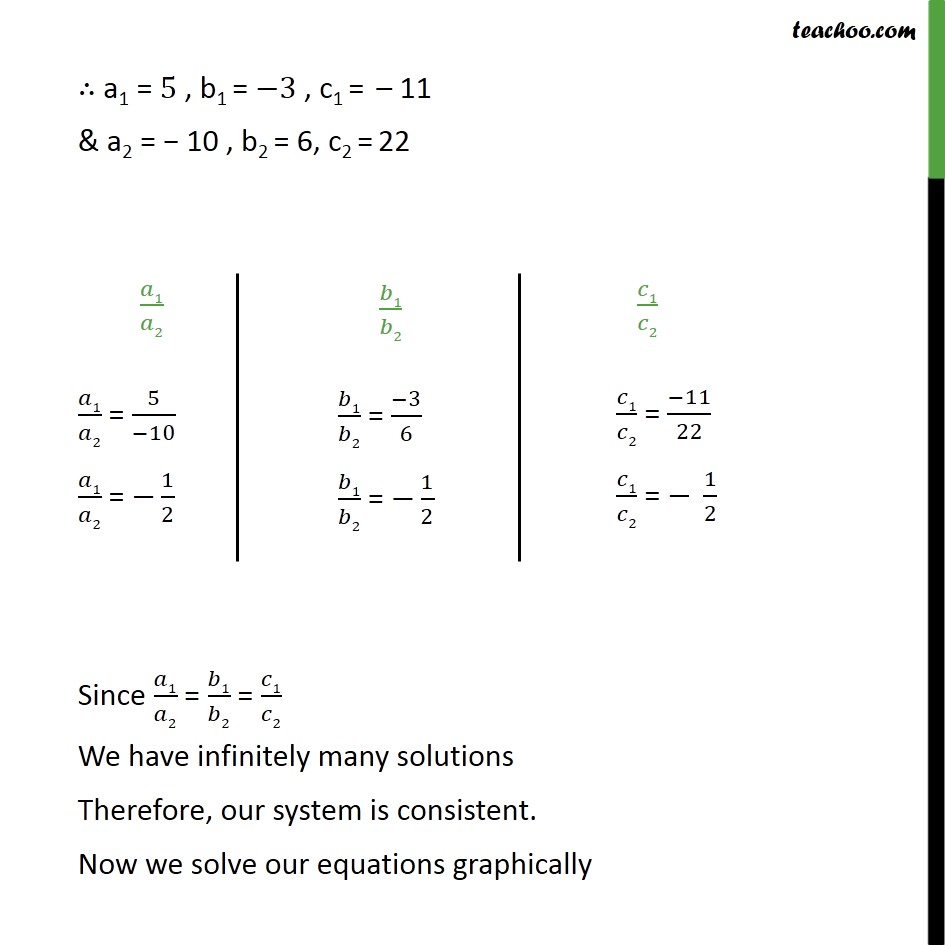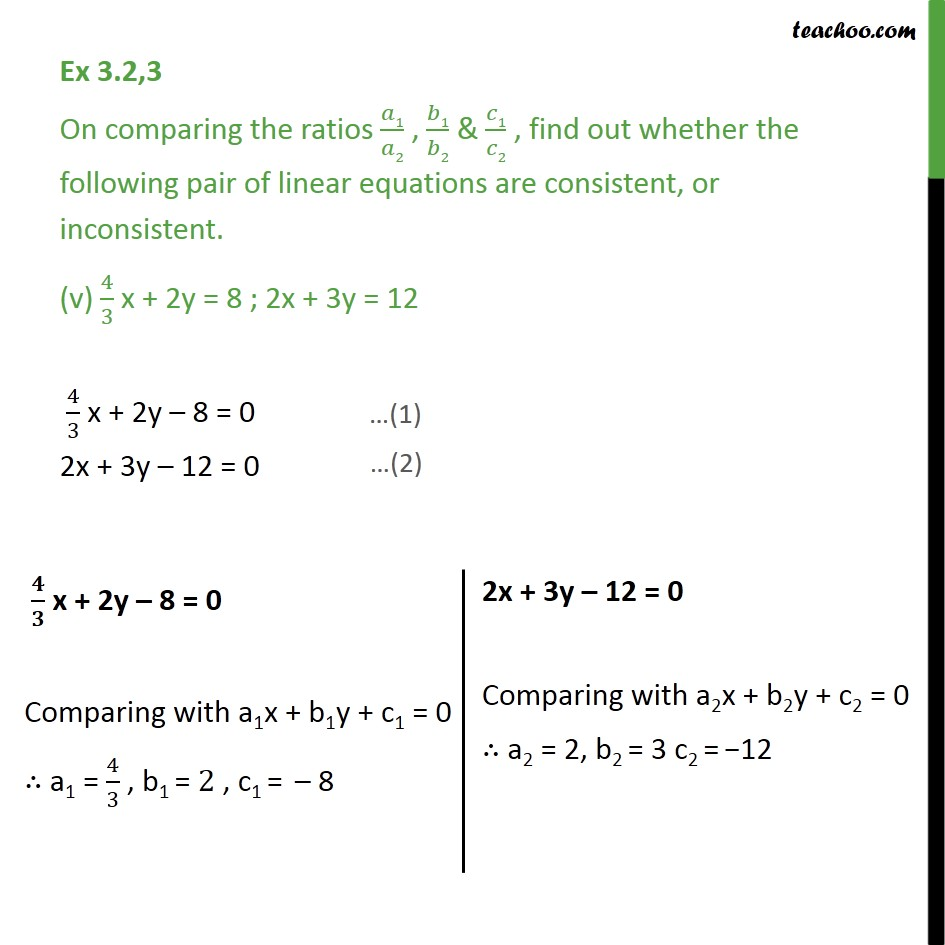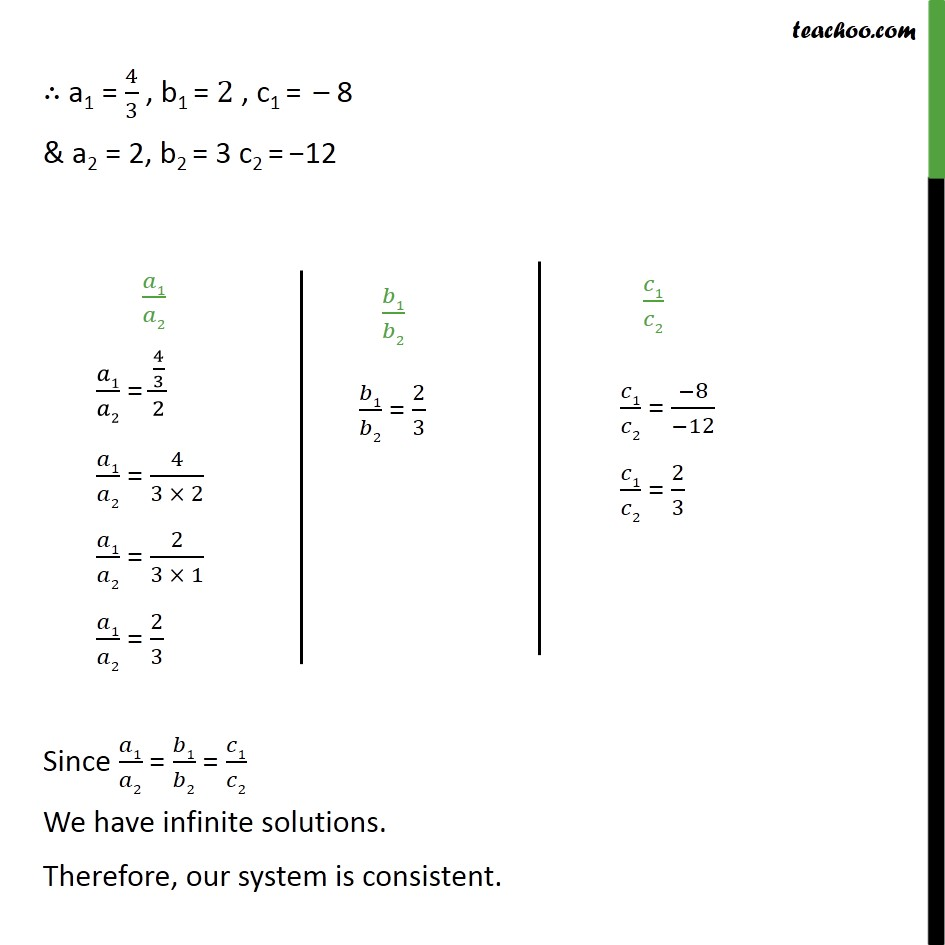1. Class 10
2. Important Questions for Exam - Class 10
3. Chapter 3 Class 10 Pair of Linear Equations in Two Variables

Transcript

Ex 3.2,3 On comparing the ratios 𝑎1/𝑎2 , 𝑏1/𝑏2 & 𝑐1/𝑐2 , find out whether the following pair of linear equations are consistent, or inconsistent. 3x+ 2y= 5 ; 2x– 3y= 7 3x + 2y – 5 = 0 2x – 3y – 7 = 0 3x + 2y – 5 = 0 Comparing with a1x + b1y + c1 = 0 ∴ a1 = 3 , b1 = 2 , c1 = –5 2x – 3y – 7 = 0 Comparing with a2x + b2y + c2 = 0 ∴ a2 = 2 , b2 = –3 , c2 = –7 a1 = 3 , b1 = 2 , c1 = –5 & a2 = 2 , b2 = –3 , c2 = –7 Since 𝑎1/𝑎2 ≠ 𝑏1/𝑏2 We have a unique solution Therefore, our system is consistent. Ex 3.2,3 On comparing the ratios 𝑎1/𝑎2 , 𝑏1/𝑏2 & 𝑐1/𝑐2 , find out whether the following pair of linear equations are consistent, or inconsistent. (ii) 2x -3y = 8 ; 4x - 6y = 9 2x – 3y − 8 = 0 4x – 6y – 9 = 0 2x – 3y − 8 = 0 Comparing with a1x + b1y + c1 = 0 ∴ a1 = 2 , b1 = − 3 , c1 = – 8 4x – 6y – 9 = 0 Comparing with a2x + b2y + c2 = 0 ∴ a2 = 4 , b2 = – 6 , c2 = – 9 ∴ a1 = 2, b1 = − 3 , c1 = − 8 a2 = 4, b2 = −6, c2 = − 9 Since 𝑎1/𝑎2 = 𝑏1/𝑏2 ≠ 𝑐1/𝑐2 We have no solution. Therefore, our system is inconsistent. Ex 3.2,3 On comparing the ratios 𝑎1/𝑎2 , 𝑏1/𝑏2 & 𝑐1/𝑐2 , find out whether the following pair of linear equations are consistent, or inconsistent. (iii) 3/2x + 5/3y = 7 ; 9x - 10y = 14 3/2 𝑥 + 5/3 𝑦 – 7 =0 9x – 10y – 14 = 0 𝟑/𝟐 𝒙 + 𝟓/𝟑 𝒚 – 𝟕 =𝟎 Comparing with a1x + b1y + c1 = 0 ∴ a1 = 3/2 , b1 = 5/3 , c1 = – 7 9x – 10y – 14 = 0 Comparing with a2x + b2y + c2 = 0 ∴ a2 = 9 , b2 = – 10, c2 = – 14 ∴ a1 = 3/2 , b1 = 5/3 , c1 = – 7 & a2 = 9 , b2 = – 10, c2 = – 14 𝒂𝟏/𝒂𝟐 𝑎1/𝑎2 = (3/2)/9 𝑎1/𝑎2 = 3/(2 × 9) 𝑎1/𝑎2 = 1/(2 ×3) 𝑎1/𝑎2 = 1/6 𝒃𝟏/𝒃𝟐 𝑏1/𝑏2 = (5/3)/10 𝑏1/𝑏2 = 5/(3 ×10) 𝑏1/𝑏2 = 1/(3 × 2) 𝑏1/𝑏2 = 1/6 𝒄𝟏/𝒄𝟐 𝑐1/𝑐2 = (−7)/(−14) 𝑐1/𝑐2 = 1/2 Since 𝑎1/𝑎2 = 𝑏1/𝑏2 ≠ 𝑐1/𝑐2 We have no solution. Therefore, our system is inconsistent. Note: This answer does not match the book answer. If we are wrong, please comment below

Chapter 3 Class 10 Pair of Linear Equations in Two Variables

Class 10
Important Questions for Exam - Class 10